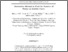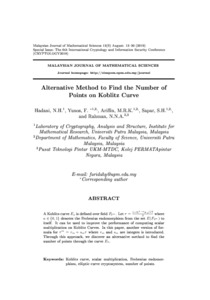# Alternative method to find the number of points on Koblitz curve

## Citation

Hadani, Nurul Hafizah and Yunos, Faridah and Kamel Ariffin, Muhammad Rezal and Sapar, Siti Hasana and Nek Abd Rahman, Normahirah (2019) Alternative method to find the number of points on Koblitz curve. Malaysian Journal of Mathematical Sciences, 13 (spec. Aug.). pp. 13-30. ISSN 1823-8343; ESSN: 2289-750X

## Abstract

A Koblitz curve Ea is defined over field F2m. Let τ = (-1)1-a+√-7/2 where a ∈ {0, 1} denotes the Frobenius endomorphism from the set E(F2m) to itself. It can be used to improve the performance of computing scalar multiplication on Koblitz Curves. In this paper, another version of formula for τ m = rm + smτ where rm and sm are integers is introduced. Through this approach, we discover an alternative method to find the number of points through the curve Ea.Preview
Text
2.pdfView Item